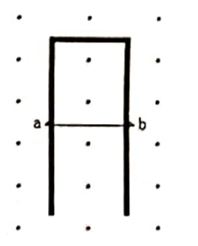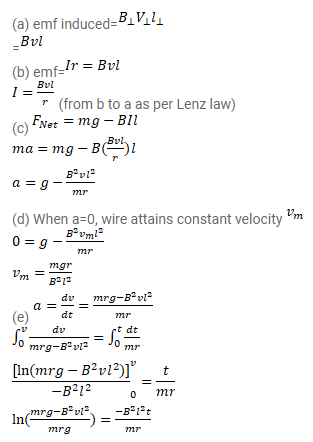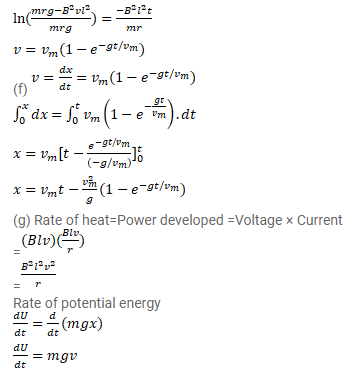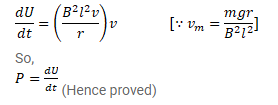# A conducting wire ab of length l,

Question:

A conducting wire ab of length $\mathrm{l}$, resistance $\mathrm{r}$ and mass $\mathrm{m}$ starts sliding at $\mathrm{t}=0$ down a smooth, vertical, thick pair of connected rails as shown in figure. A uniform magnetic field $B$ exists in the space in a direction perpendicular to the plane of the rails.

(a) Write the the induced emf in the loop at an instant $t$ when the speed of the wire is v.

(b) What would be the magnitude and direction of the induced current in the wire?

(c) Find the downward acceleration of the wire at this instant.

(d) After sufficient time, the wire stats moving with a constant velocity. Find this velocity $v_{m}$,

(e) Find the velocity of the wire as a function of time.

(f) Find the displacement of the wire as a function of time.

(g) Show that the rate at which the gravitational potential energy is decreased after steady state is reached.Solution: